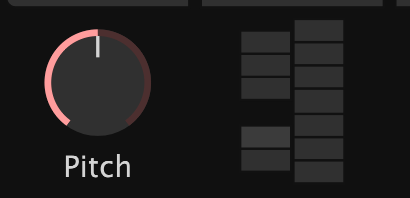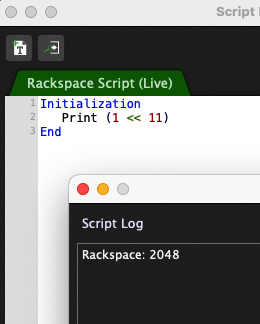# MIDI input to Bitwise Operation?

New GP user, really loving the app, having a ton of fun building performance rigs, so much more intuitive than any other DAW/etc.

I’m currently building a setup primarily focused around the Emergence VST, a granular synthesis effect. The plug-in has a GUI parameter that looks like a keyboard that you use to set the note quantization for the pitch parameter. Basically, one octave of a keyboard:I had hoped that each key of the “keyboard” would be a different VST parameter so they could be individually turned on/off with widgets. But it seems like the whole keyboard is a single parameter, with a 4 digit value, where each value is a different combination of activated keys. I reached out to the developer and he gave me this explanation:

The states for all the pitch buttons are encoded as a single number.
The numeric value you see makes more sense if you think of it as an 11-bit binary number with each switch assigned to a different bit.
The lowest switch on the UI (C on the piano keyboard) will toggle the lowest (least significant) bit, adding 2^0 = 1 (two to the power of 0). The next button (C#) toggles the next bit, adding 2^1=2 to the parameter value when toggled on.
And so on up to 2^11=2048 for the topmost button.
It should be possible in a script to read incoming midi notes and set the corresponding bits using bitwise operations and send out the result as a single CC value that is mapped to the parameter.

My scripting skills are not great but also not non-existant, so I could probably take a stab at it. Other than RTM, I just want to make sure bitwise operations were possible with GP4 and if there were any tips or guides you guys would recommend as a starting point for figuring this out?

Thanks!

RTM is in fact the appropriate solution - that’s why we wrote it2 Likes

2048 needs 12 bits. Makes sense when you realize that one octave has 12 notes

2 Likes

Yeah, I had a feeling that part was a typo.

I’ll report back how far I get.

OK, I’m close. Here’s what I got:

``````Var
EmV1Scale : Widget
HapaxInput : MidiInBlock

On NoteEvent(m : NoteMessage) from HapaxInput
Var
ScaleValue : Integer
thisNote : Integer

SendNow(HapaxInput, m)

thisNote = GetNoteNumber(m)

If IsNoteOn(m)
then
If thisNote == 60 Or thisNote == 72 then ScaleValue = ScaleValue + 1 else
If thisNote == 61 Or thisNote == 73 then ScaleValue = ScaleValue + 2 else
If thisNote == 62 Or thisNote == 74 then ScaleValue = ScaleValue + 4 else
If thisNote == 63 Or thisNote == 75 then ScaleValue = ScaleValue + 8 else
If thisNote == 64 Or thisNote == 76 then ScaleValue = ScaleValue + 16 else
If thisNote == 65 Or thisNote == 77 then ScaleValue = ScaleValue + 32 else
If thisNote == 66 Or thisNote == 78 then ScaleValue = ScaleValue + 64 else
If thisNote == 67 Or thisNote == 79 then ScaleValue = ScaleValue + 128 else
If thisNote == 68 Or thisNote == 80 then ScaleValue = ScaleValue + 256 else
If thisNote == 69 Or thisNote == 81 then ScaleValue = ScaleValue + 512 else
If thisNote == 70 Or thisNote == 82 then ScaleValue = ScaleValue + 1024 else
If thisNote == 71 Or thisNote == 83 then ScaleValue = ScaleValue + 2048 else
End End End End End End End End End End End End
Else
If thisNote == 60 Or thisNote == 72 then ScaleValue = ScaleValue - 1 else
If thisNote == 61 Or thisNote == 73 then ScaleValue = ScaleValue - 2 else
If thisNote == 62 Or thisNote == 74 then ScaleValue = ScaleValue - 4 else
If thisNote == 63 Or thisNote == 75 then ScaleValue = ScaleValue - 8 else
If thisNote == 64 Or thisNote == 76 then ScaleValue = ScaleValue - 16 else
If thisNote == 65 Or thisNote == 77 then ScaleValue = ScaleValue - 32 else
If thisNote == 66 Or thisNote == 78 then ScaleValue = ScaleValue - 64 else
If thisNote == 67 Or thisNote == 79 then ScaleValue = ScaleValue - 128 else
If thisNote == 68 Or thisNote == 80 then ScaleValue = ScaleValue - 256 else
If thisNote == 69 Or thisNote == 81 then ScaleValue = ScaleValue - 512 else
If thisNote == 70 Or thisNote == 82 then ScaleValue = ScaleValue - 1024 else
If thisNote == 71 Or thisNote == 83 then ScaleValue = ScaleValue - 2048 else ScaleValue = 0
End End End End End End End End End End End End
End

Print(ScaleValue)
SetWidgetValue(EmV1Scale, ScaleValue)
End
``````

I’m sure there’s a more elegant way to do this, but in the Script Logger I am seeing the value I want.

However, the problem is the Widget. If I adjust the widget with my mouse, I can see the value scrolling from 0 to 4095 as expected from the plugin. But when I use my script any key I press on my controller causes the widget to jump to 4095. I’m guessing it’s a scaling issue? Integer vs double? What’s the correct way to input my ScaleValue variable into the value of the Widget?

Thanks!

Quick and dirty:

``````On NoteEvent(m : NoteMessage) from HapaxInput
Var
ScaleValue : Integer = 0
thisNote : Integer = 0

SendNow(HapaxInput, m)

thisNote = GetNoteNumber(m)

if IsNoteOn(m)
then
ScaleValue = Floor(Power(2, (thisNote % 12)))
else
ScaleValue = - Floor(Power(2, (thisNote % 12)))
end

Print("ScaleValue option A: " + ScaleValue)
End
``````

More efficient:

``````var pwrs : integer

Initialization
var i : integer = 0

for i=0; i<16; i = i + 1 do
pwrs[i]=Floor(Power(2, i))
end
End

On NoteEvent(m : NoteMessage) from HapaxInput
Var
ScaleValue : Integer = 0
thisNote : Integer = 0

SendNow(HapaxInput, m)

thisNote = GetNoteNumber(m)

if IsNoteOn(m)
then
ScaleValue = pwrs[thisNote % 12]
else
ScaleValue = - pwrs[thisNote % 12]
end

Print("ScaleValue option B: " + ScaleValue)
End
``````

The second option should be more efficient, because Power(,) is rather expensive, but the first option is more self-contained

I did not try this out, so you have to check, but it should at least compile

Edit: corrected the initialization.

2 Likes

Thanks a bunch, these both work with one slight modification: in order to keep track of which keys have been pressed then let go, ScaleValue needs to be initialized as 0 and then after that ScaleValue needs to be added to itself to keep track of what’s on/off. Taking the first example for ease of seeing the tweaks:

``````Var
EmV1Scale : Widget
HapaxInput : MidiInBlock
ScaleValue : Integer
pValue : double

Initialization

ScaleValue = 0

End

On NoteEvent(m : NoteMessage) from HapaxInput
Var
thisNote : Integer = 0

SendNow(HapaxInput, m)

thisNote = GetNoteNumber(m)

if IsNoteOn(m)
then
ScaleValue = ScaleValue + Floor(Power(2, (thisNote % 12)))
else
ScaleValue = ScaleValue - Floor(Power(2, (thisNote % 12)))
end

//    pValue = GetWidgetValue(EmV1Scale)
//    Print(pValue)
//        Print(1.0/4095.0)

Print(ScaleValue)
Print(ScaleValue * (1.0/4095.0))
SetWidgetValue(EmV1Scale, ScaleValue * (1.0/4095.0))
End
``````

Thanks for the two cleaner options, also feeling good that I was able to figure out how to have the value cumulative as keys are pressed.

But I’m still at a roadblock for getting the Widget to receive the correct value from the script. I realized that while the Widget might display the 4 digit number from the VST, the actual value is a float from 0.0 to 1.0, so I have to scale that number. So, I multiplied the ScaleValue by 0.0002442, which is 1/4095. This gets me close, but there are still issues. If I send it small numbers like 1 (aka 0.0002442) the Widget value does not change. But once I get to 4 and higher it does, but sometimes the numbers aren’t correct. Like sending the value 64 from the script displays as 66 on the widget.

I think it’s a resolution issue. If I adjust the parameter in the VST to, say, 1 the widget will display 1. If I then use a GetWidgetValue function and print it to the Logger, instead of seeing 0.0002442 I instead see 0.0010000. Can GScript handle values this small or is it rounding these smaller values? Same happens when I try

Right. ‘thisNote’ I should have initialized to for example 61 to test my code. ScaleValue and thisNote must be initalized to ‘0’. Sorry. As I said, I couldn’t really test it, but you’ve corrected it1 Like

This could be a problem when 2 noteon-s arrive in various octaves, before a noteoff comes in. Tonight I will look into that using a real bitwise approach

1 Like

The bitwise approach:

``````On NoteEvent(m : NoteMessage) from ....
Var
thisNote : Integer = 0

SendNow(local, m)

thisNote = GetNoteNumber(m)

if IsNoteOn(m)
then
ScaleValue = ScaleValue or Floor(Power(2, (thisNote % 12)))
else
ScaleValue = ScaleValue and not Floor(Power(2, (thisNote % 12)))
end
Print("SV: " + ScaleValue)
// ToDo:
// SetWidgetValue(...)
End
``````
1 Like

I had thought of that, but was planning on dealing with it by simply being thoughtful when playing my MIDI controller, but your bitwise solution works perfectly!! Many thanks!

For anyone curious, I figured out how to get around this issue. Instead of using SetWidgetValue I went straight to the plugin instead with SetParameter. Then there was no resolution issue as with the Widget.

Here’s the final script (with a little channel selection thrown in) for anyone else that wants to do something similar, fully recognition going to @Frank1119 for the heavy lifting:

``````Var
HapaxInput : MidiInBlock
ScaleValue : Integer
EmVoice1 : PluginBlock

Initialization

ScaleValue = 0

End

On NoteEvent(m : NoteMessage) from HapaxInput

Var
thisNote : Integer = 0

SendNow(HapaxInput, m)

thisNote = GetNoteNumber(m)

if IsNoteOn(m)
then
ScaleValue = ScaleValue or Floor(Power(2, (thisNote % 12)))
else
ScaleValue = ScaleValue and not Floor(Power(2, (thisNote % 12)))
end

if GetChannel(m) == 1
then
SetParameter(EmVoice1,18, ScaleValue *(1.0/4095.0))
end

End
``````

I’m a little confused — what is the purpose of the Power function?

It is a bitmapped value so to set bit 0 an ‘or’ with 0 is necessary, to set bit 11 an ‘or’ with 2048 us needed. The notes for one octave however are numbered 60, 61 … 71, for another octave 72,73 … 83. So to get the right value for the bit to set power(2, 12) → 2048. For example note c == 60. Notenumber = 60 % 12 (== 0). Power(2,0) == 0, so ScaleValue’s lsb must be set (ScaleValue = ScaleValue or 1)

Yes, I understand that — but why do you need the Power function to do that? Why can’t you just write

1 << 11 // Shift 11 bits

For example:1 Like

Yes. That’s the better option. Just didn’t know that the shift operator is available.

I’ll revise my answer and credit you also, but it will have to wait a few hours. I’m at the office now1 Like

Mmm, that’s why we have this thing called a language manual for GP Script and in particular,

It’s worth readingI did several times, but I’m using a lot of different platforms, so I’m not always remembering the features of each platform.Not an excuse: an explanation

1 Like

I’m not sure about different platforms but this feature is in the official release 4.58

Are you using a version older than 4.58?

Platforms as in bash, c#, c++, python, lua, gpscript, powershell, etc.

When it comes to GP, solely 4.5.8 for ‘production code’

1 Like